Self-avoiding polymer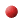Simulations: The dimerization method was used to generate samples of 108 SAWs on a cubic lattice,

for N =16, 32,...,1024.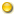By measuring the probability that a generated SAW does not intersect the boundary, we  obtain N-Δγ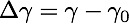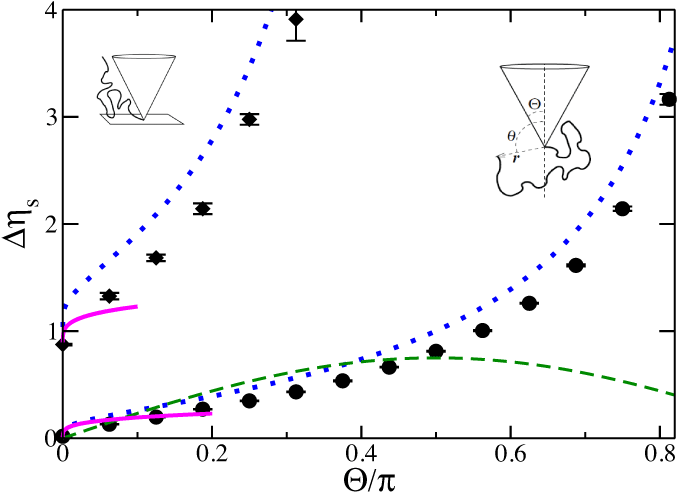The phantom polymer results provide an excellent approximation, except for very thin cones.The green dashed line is from an ε-expansion, treating the surface of the cone as a weak perturbation:

"Apex exponents for polymer-probe interactions,"

M. Slutsky, R. Zandi, Y. Kantor, M. Kardar, PRL 94, 198303 (2005)ε-expansion: A better treatment is to start with impenetrable boundaries,

along the lines of a similar computation for a wedge

"Critical behaviour at an edge," J.L. Cardy, J. Phys. A: Math. Gen. 16 3617

"Polymer-mediated entropic forces between scale-free objects,"

M. F. Maghrebi, Y. Kantor, M. Kardar, PRE 86, 061801 (2012)The loop-correction can only be calculated numerically in general, but for small opening angles we find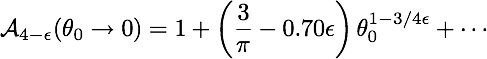Is the exponent for the singular dependence on angle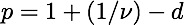?Current simulations are not sufficient to answer this question